Courses

# Past Year Questions: Footing, Columns, Beams & Slabs Notes | EduRev

## Civil Engineering (CE) : Past Year Questions: Footing, Columns, Beams & Slabs Notes | EduRev

The document Past Year Questions: Footing, Columns, Beams & Slabs Notes | EduRev is a part of the Civil Engineering (CE) Course Topic wise GATE Past Year Papers for Civil Engineering.
All you need of Civil Engineering (CE) at this link: Civil Engineering (CE)

Q.1 A singly-reinforced rectangular concrete beam of width 300 mm and effective depth 400 mm is to be designed using M25 grade concrete and Fe500 grade reinforcing steel. For the beam to be underreinforced, the maximum number of 16 mm diameter reinforcing bars that can be provided is    [2018 : 2 Marks, Set-II]
(a) 3
(b) 4
(c) 5
(d) 6
Ans.
(C)
Solution:
B = 300 mm
d = 400 mm (effective depth)
M25 and Fe500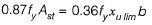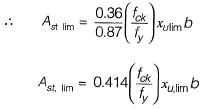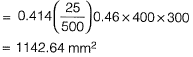Number of 16 mm φ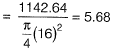For Ast < Ast lim, maximum number of bars to be provided is 5 to keep the beam as underreinforced section. If the reinforcement provided exceed 5.68 No. (say 6 and more) the section will become over-reinforced.

Q.2 A structural member subjected to compression, has both translation and rotation restrained at one end, while only translation is restrained at the other end. As per IS 456 : 2000, the effective length factor recommended for design is    [2018 : 1 Mark, Set-II]
(a) 0.50
(b) 0.65
(c) 0.70
(d) 0.80
Ans.
(D)
Solution:
One end is fixed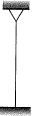Other end is pin jointed
Effective length of column (as per IS:456- 2000)
= 0.80 L

Q.3  A reinforced-concrete slab with effective depth of 80 mm is simply supported at two opposite ends on 230 mm thick masonry walls. The centre-to- centre distance between the walls is 3.3 m. As per IS 456 : 2000, the effective span of the slab (in m, up to two decimal places) is __________ .    [2018 : 1 Mark, Set-II]
Solution: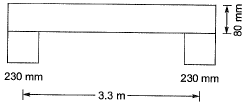Here support width = 230 mm
Effective depth of slab = 80 mm
Clear span = 3.3 - 0.23 = 3.07 m
Effective span = Min. of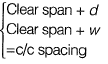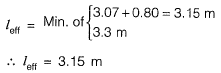Q.4 An RCC short column (with lateral ties) of rectangular cross-section of 250 mm x 300 mm in reinforced with four members of 16 mm diameter longitudinal bars. The grades of steel and concrete are Fe415 and M20, respectively. Neglect eccentricity effect. Considering limit state of collapse in compression (IS 456 : 2000), the axial load carrying capacity of the column (in kN, up to one decimal place) is _____.    [2018 : 2 Marks, Set-I]
Solution:

Since eccentricity effect is being neglected so column can be considered as concentrically loaded. Ultimate axial load carrying capacity of column.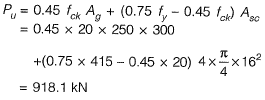Q.5 Two rectangular under-reinforced concrete beam sections X and Yare similar in all aspects except that the longitudinal compression reinforcement in section Y is 10% more. Which one of the following is the correct statement?    [2018 : 1 Mark, Set-I]
(a) Section X has less flexural strength and is less ductile than section Y
(b) Section X has less flexural strength but is more ductile than section Y
(c) Section X and Y have equal flexural strength but different ductility
(d) Sections Xand Y have equal flexural strength and ductility.
Ans.
(A)
Solution: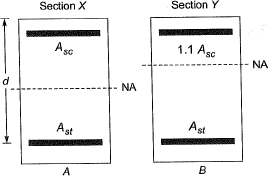Due to presence ot more compression steel in section Y, NA of section of Xis above than as of X. It means Yis more under-reinforced than X so ductility of Y is more.
Since compression steel of Yis more so flexure resistance of X is less than as of Y.

Q.6  A reinforced concrete (RC) beam with width of 250 mm and effective depth of 400 mm is reinforced with Fe 415 steel. Consider 50 mm effective cover. As per the provisions of IS 456 : 2000, the minimum and maximum amount of tensile reinforcement (expressed in mm2) for the section are, respectively    [2016 : 2 Marks, Set-I]
(a) 250 and 3500
(b) 205 and 4500
(c) 270 and 2000
(d) 300 and 2500
Ans.
(B)
Solution:
Minimum tension reinforcement is given by,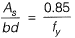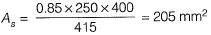Maximum compression reinforcement = 0.04 bD
= 0.04 x 250 x 450
= 4500 mm2

Q.7 A haunched (varying depth) reinforced concrete beam is simply supported at both ends, as shown in the figure. The beam is subjected to a uniformly distributed factored load of intensity 10 kN/m. The design shear force (expressed in kN) at the section X-X of the beam is_______ . (Neglect variation of load due to change in section)    [2016 : 2 Marks, Set-Il]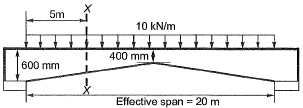Solution: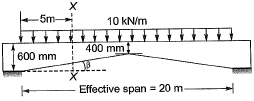Shear force at section X-X,
Vu = 100 - 5 x 10 = 50 kN
Depth at section X-X,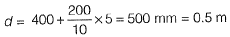Moment at section X-X,
MU = 100 x 5 - 10 x 2.5 x 5 = 375 kNm
Design shear force at section X-X,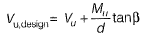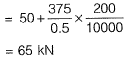Q.8 A column of size 450 mm x 600 mm has unsupported length of 3.0 m and is braced against side sway in both directions. According to IS 456: 2000, the minimum eccentricities (in mm) with respect to major and minor principle axes are    [2015 : 1 Mark, Set-Il]
(a) 20.0 and 20.0
(b) 26.0 and 21.0
(c) 26.0 and 20.0
(d) 21.0 and 15.0
Ans.
(B)
Solution: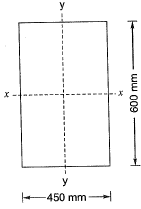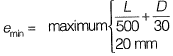x-x will be major axis and y-y will be minor axis.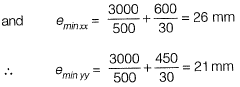Q.9 For a beam of cross-section, width = 230 mm and effective depth = 500 mm, the number of rebars of 12 mm diameter required to satisfy minimum tension reinforcement requirement specified by IS 456 : 2000 (assuming grade of steel reinforcement as Fe500) is ________ .    [2014 : 2 Marks, Set-I]
Solution: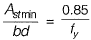or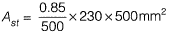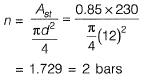Q.10 The cross-section at mid-span of a beam at the edge of a slab is shown in the sketch. A portion of the slab is considered as the effective flange width for the beam. The grades of concrete and reinforcing steel are M25 and Fe415 respectively. The total area of reinforcing bars (As) is 4000 mm2. At the ultimate limit sate, xu denotes the depth of the neutral axis from the top fibre. Treat the section as under-reinforced and flanged (xu >100 mm).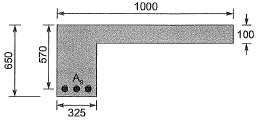The ultimate moment capacity (in kNm) of the sections as per the Limit State Method of IS 456 : 2000 is    [2012 : 2 Marks]
(a) 475.2
(b) 717.0
(c) 756.4
(d) 762.5
Ans.
(B)
Solution: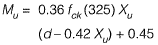or,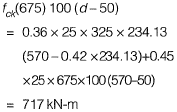Take 717.00 kNm

Q.11 The cross-section at mid-span of a beam at the edge of a slab is shown in the sketch. A portion of the slab is considered as the effective flange width for the beam. The grades of concrete and reinforcing steel are M25 and Fe415 respectively. The total area of reinforcing bars (As) is 4000 mm2. At the ultimate limit sate, xu denotes the depth of the neutral axis from the top fibre. Treat the section as under-reinforced and flanged (xu >100 mm).The value of xu(in mm) computed as per the Limit State Method of IS 456 : 2000 is    [2012 : 2 Marks]
(a) 200.0
(b) 223.3
(c) 236.3
(d) 273.6
Ans.
(C)
Solution:Given, Xu > 100 mm and section as under reinforced as per IS 456 : 2000
Effective width of flange of L-beam: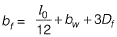Where l0 = Distance between point of zero moment in beam
Df = Depth of flange
bw = Width of web
Taking bf = 1000 mm
∴ Length of beam is not given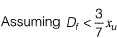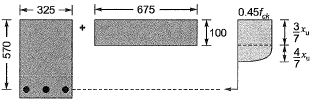∴ Equating compressive force and tensile force
C = T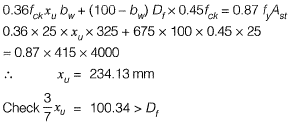It shows our assumption was correct.
So, xu = 234.13 mm
(Nearest match = 236 mm)

Q.12 A doubly reinforced rectangular concrete beam has a width of 300 mm and an effective depth of 500 mm. The beam is reinforced with 2200 mm2 of steel in tension and 628 mm2 of steel in compression. The effective cover for compression steel is 50 mm. Assume that both tension and compression steel yield. The grades of concrete and steel used are M20 and Fe250, respectively. The stress block parameters (rounded off to first two decimal places) for concrete shall be as per IS 456 : 2000.
A 16 mm thick plate measuring 650 mm x 420 mm is used as a base plate for an ISHB 300 column subjected to a factored axial compressive load of 2000 kN. As per IS 456 : 2000, the minimum grade of concrete that should be used below the base plate for safely carrying the load is    [2011 : 1 Mark]
(a) M15
(b) M20
(c) M30
(d) M40
Ans.
(B)
Solution: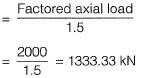The developed bearing pressure on concrete is given as,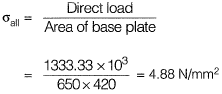The developed stress in direct compression in various grades of concrete as per IS 456 : 2000 are tabulated below = 0.25 fck (WSM)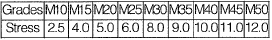The permissible stress in concrete should be more than the developed bearing pressure. Thus the minimum grade of concrete which should be used is M 20.

Q.13 A doubly reinforced rectangular concrete beam has a width of 300 mm and an effective depth of 500 mm. The beam is reinforced with 2200 mm2 of steel in tension and 628 mm2 of steel in compression. The effective cover for compression steel is 50 mm. Assume that both tension and compression steel yield. The grades of concrete and steel used are M20 and Fe250, respectively. The stress block parameters (rounded off to first two decimal places) for concrete shall be as per IS 456 : 2000.
The moment of resistance of the section is
(a) 206.00 kN-m
(b) 209.20 kN-m
(c) 237.80 kN-m
(d) 251.90 kN-m
Ans.
(B)
Solution:
Moment of resistance Mu is given by,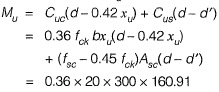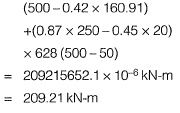Q.14 A doubly reinforced rectangular concrete beam has a width of 300 mm and an effective depth of 500 mm. The beam is reinforced with 2200 mm2 of steel in tension and 628 mm2 of steel in compression. The effective cover for compression steel is 50 mm. Assume that both tension and compression steel yield. The grades of concrete and steel used are M20 and Fe250, respectively. The stress block parameters (rounded off to first two decimal places) for concrete shall be as per IS 456 : 2000.    [2010 : 2 Marks]
The depth of neutral axis is
(a) 205.30 mm
(b) 184.56 mm
(c) 160.91mm
(d) 145.30 mm
Ans. (C)
Solution: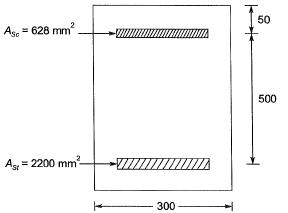The resultant compressive force in the concrete is given by,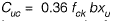The resultant compressive force in the compression steel is given by,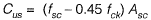The resultant tensile force in the tensile steel is given by,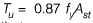we know that,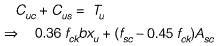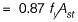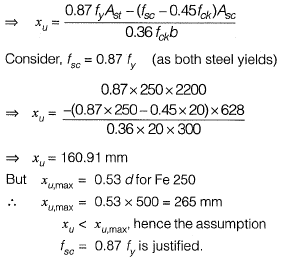Offer running on EduRev: Apply code STAYHOME200 to get INR 200 off on our premium plan EduRev Infinity!

67 docs

,

,

,

,

,

,

,

,

,

,

,

,

,

,

,

,

,

,

,

,

,

,

,

,

,

,

,

;